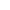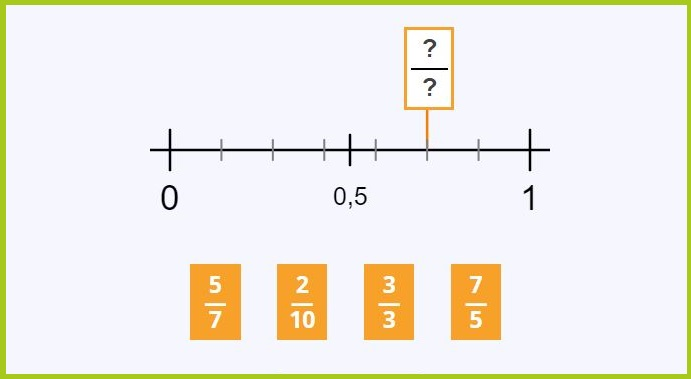﻿ Fractions on a Number Line Games + Worksheets

Page No. 1032

# Fractions on a number line games

Locate fractions on a number line games online. Select the fraction that corresponds to the given point on the number line. Placing fractions on a number line´ ONLINE games. Fraction number line 0 to 1. Free printable fractions on a numberline worksheets PDF. Placing fractions on a number line PRACTICE. fraction games on a number line. teaching fractions on a number line activities. Missing fractions on a number line game. interactive fraction number line. 0 to 1 number line fractions.

New gameSelect the fraction that corresponds to the point on the number line.

## Locate fractions on a number line GAMES"Fractions on a Number Line" is an online game that is designed to help kids understand fractions and their relationship to a number line.

### Fraction line game

The "fraction line" game presents a number line and a specific point on that line, and then offers 4 different fraction options to choose from. You must correctly select the fraction that corresponds to the point on the number line. Press the button "New game" and you can play a new fraction numberline game.

## Free printable fractions on a numberline worksheets PDF

Click the image bellow and generate endless number of free printable fractions on a numberline worksheets in PDF. These printable fractions on a number line activities include an answer key on the other page.

You can find more fractions worksheets here.

## Missing fractions on a number line: GAME free

The fraction line game is an user-friendly interactive fraction game, with clear graphics and easy-to-use controls. Students can select their answers by clicking on the appropriate fraction option, and the game will immediately provide feedback, indicating whether the answer was correct or incorrect.

While playing the fractions on a number line game, players can practice their knowledge of fractions, including identifying fractions and understanding how to locate fractions on a number line.## 0 to 1 Number line with fractions

A number line is a line that represents numbers of equal intervals. A fraction number line is a visual representation of fractions arranged in a linear fashion. Our fraction line consists of a horizontal line with zero on the left side, and number 1 on the right side.

### Fractions on a number line GAMES

Fraction lines are divided into equal parts. Usually, the denominator of the fraction represents the total number of equal parts in the whole, and the numerator represents the number of parts being considered.

For example, if the whole is divided into four equal parts, and three of those parts are being considered, then the fraction would be 3/4.

### Fraction number line 0 to 1

Our fraction number line is sometimes divided to tenths but sometimes to parts as the denominator.

Using the denominator to divide the number line can be a good option for younger students who are just learning about fractions, as it provides a simple, uniform scale to work with. This can help them develop a basic understanding of how fractions are represented on a number line and how they relate to each other.

On the other hand, dividing the number line into tenths can be a good option for older or more advanced students who are working with more complex fractions. This can help them see the relationship between the fraction's denominator and the size of the parts represented on the number line, as well as providing a more precise scale for measuring and converting fractions to decimals.

Ultimately, the decision to divide the number line into tenths or into parts matching the denominator is made randomly in this fractions on a number line GAME.

## Fraction number line 0 to 1

When the number line ranges from 0 to 1, the player is presented with fractions that are between 0 and 1, it means proper fractions, where the numerator is less than the denominator.

## Interative fraction number line activities

One additional point to consider is the importance of feedback when using games and worksheets to teach fractions on a number line. Feedback is crucial in helping students understand their mistakes and correct them, as well as providing positive reinforcement for correct answers.

In this online game "Fractions on a Number Line," feedback is be provided through immediate feedback after each question, including whether the answer is correct or incorrect. In fractions n a number line worksheets, feedback is provided through self-checking mechanisms in the anwer key.

## Placing fractions on a number line PRACTICE

Fraction number lines are an effective tool for teaching and learning fractions because they provide a visual representation of fractions and their relationship to whole numbers. Here are some ways in which fraction lines can be helpful for learning fractions:

### Benefits of fraction games on a number line

• Visual representation: 💡 When kids are already familiar how fractions look like, for example through fraction flash cards, they can continue to visualize fractions as points on a fraction line. This help students understand the concept of fractions as representing a part of a whole.
• Comparing fractions: 💡 When you place fractions on a number line, it is easier to compare and order them. Students can see which fractions are greater or smaller, and how equivalent fractions are related.
• Ordering fractions: 💡 Number lines can be used to order fractions by placing them in order from least to greatest or greatest to least. This helps students understand how to compare and order fractions.
• Adding and subtracting fractions: 💡 Number lines can be used to add and subtract fractions by finding a common denominator and then locating the fractions on the line to determine the sum or difference.
• Identifying equivalent fractions: 💡 Number lines are used to identify equivalent fractions because they are placed at the same point on the line. This helps students understand that different fractions can represent the same quantity.

## Fractions on a number line online game

In the game "Fractions on a Number Line," the player is presented with a number line and a specific point on that line, and they must select the fraction that corresponds to that point. You can learn how to place fractions on a number line on YouTube here.

The game helps players develop their understanding of fractions by visualizing them on a number line and allowing them to see how fractions relate to other fractions and whole numbers.

Category: